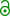## Science vs. (EMA) GL aka PK primer [Regulatives / Guidelines]

Dear all,

replying myself to clarify things. Starter: Scheerans et al. (2008).1 tmax in a monoexponential model is the root of the first derivative of the concentration-time curve and given by

$$\small{t_{max}=\log(k_a / k_{el}) / (k_a-k_{el})}$$

The inflection point tinpt where the sign of the curvature changes (or the tangent moves from above to below the curve) is the root of the second derivative and given by

$$\small{t_{inpt}=2\cdot \log(k_a / k_{el}) / (k_a-k_{el})}$$

If you want to play around yourself I suggest to convert the Bateman-function (with volume, fraction absorbed, and rate constants) to a sum of expo­nen­tials, i.e.,

$$\small{f(x)=A(e^{-k_{el}\cdot x}-e^{-k_a\cdot x})}$$.

The first and second derivatives are

$$\small{f'(x)=A(-k_{el}\cdot e^{-k_{el}\cdot x}+k_a\cdot e^{-k_a\cdot x})}$$ and
$$\small{f''(x)=A(k{_{el}}^{2}\cdot e^{-k_{el}\cdot x}-k_{a}^{2}\cdot e^{-k_a\cdot x})}$$.

The percentage absorbed is given by

$$\small{\%\; abs = 100(1-e^{-k_a \cdot x})}$$

AUC in the interval [0, a] is given as the definite integral of ƒ(x) by

$$\small{A \left ( (e^{k_a \cdot a}-1)/k_a - (e^{k_{el}\cdot a}-1)/k_{el} \right )}$$

Example: A 400, ka 1.3863 (t½a 0.5), kel 0.6931 (t½el 1). Although Scheerans et al. recom­mended their procedure for ka ≥ 3kel in order to avoid problems with flip-flop PK (which is unlikely for IR formulations) I used a ratio of 2 in order to get nice numbers.At tmax 75% are absorbed (AUCt/AUC 25%) and at tinpt already 93.75% (AUCt/AUC 56.25%). When do we have a ratio of 80%? At 3.244 (where 98.89% are absorbed). That’s a very, very conservative value. Note that tinpt equals four absorption half-lives (alternative method for the calculation of % absorbed: 100 – 100/24·t½a = 93.75%). If one wants to be more conservative and follow the logic of the GL (wash-out, saturation to steady state) and use five half-lives one could stop at 2.5.

tlast        % abs  % AUC∞ 1.0 = tmax   75.00  25.00 2.0 = tinpt  93.75  56.25 2.161        95.00  60.28 2.5 = 5×t½a  96.88  67.77 3.244        98.89  80.00 3.322        99.00  81.00

So why a cut-off of 80%?* Since tinpt is a reasonable starting point for the estimation of λz (forget max. R²adj including earlier time points since elimination is contaminated by absorption), it should be possible to have ≥3 data points in the interval [tinpt, tlast]. We can expect to get a reliable estimate of λz. But what’s the use of it? The EMA’s metric for extent of absorption is AUCt. We could reliably estimate it with an earlier cut-off as well!2,3 Are we comparing λz of test and reference? No. On the contrary – we assume identical inter-occasion clearances (or V + kel if you belong to the other church).
IMHO, the cut-off of 80% is scientifically not justified for monoexponential models (including ones with a lag-time: homework). Would only make sense if we would use AUCs corrected by kel,4 which seems not to be acceptable by EMA (at least). It’s strange to judge the validity of a study based on eli­mi­na­tion rather than essentially complete absorption.

Unfortunately it is not that easy for multicompartimental models – or even worse – profiles with multiple peaks, though absorption will follow the same time course. MR (CR in particular) is another cup of tea. We might be in flip-flop limbo and personally I would not use AUCt as the main metric at all. I’m curious what the draft MR GL will tell us… I will propose AUC with an extrapolated area of well below 20%.

@Ratnakar: If you want help it would be a good idea to answer all of the questions in my previous post (see the Forum’s policy).

1. Scheerans C, Derendorf H, Kloft C. Proposal for a Standardised Identification of the Mono-Exponential Terminal Phase for Orally Administered Drugs. Biopharm Drug Dispos. 2008;29:145–57. doi:10.1002/bdd.596.
2. Midha KK, Hubbard JW, Rawson MJ. Retrospective evaluation of relative extent of absorption by the use of partial areas under plasma concentration versus time curves in bioequivalence studies on conventional release products. Europ J Pharm Sci. 1996;4:381–4. doi:10.1016/0928-0987(95)00166-2.
3. Midha KK, Hubbard JW, Rawson MJ, Gavalas L. The application of partial areas in assessment of rate and extent of absorption in bioequivalence studies of conventional release products: Experimental evidence. Europ J Pharm Sci. 1994;2(5–6):351–63. doi:10.1016/0928-0987(94)00062-X.
4. Abdallah HY. An Area Correction Method To Reduce Intrasubject Variability In Bioequivalence Studies. J Pharm Pharmaceut Sci. 1998;1(2):60–5.free resource.

• Note that the FDA – although both AUCt and AUC are required – doesn’t have such a cut-off.

Dif-tor heh smusma 🖖
Helmut SchützThe quality of responses received is directly proportional to the quality of the question asked. 🚮
Science QuotesIng. Helmut Schütz